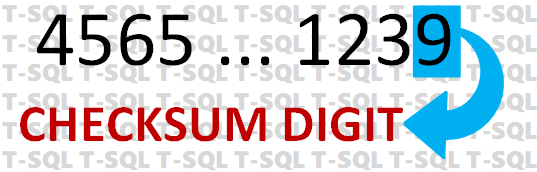Entering in long sequences of numbers can be fraught with error, say as in a sixteen-digit credit card number or a twelve-digit social security card number. A scientist at IBM in the middle of the last century came up with a formula specifically designed to avoid errors in long numbers called the Luhn Formula or Luhn Algorithm. It works by adding the individual numbers in a specific fashion and resolving that addition to a single digit that is then appended to the end of the number.By checking the last number against the preceding number using the Luhn formula, the computer can tell the operator if it is a valid number or not. There is a 10 per cent chance that an error might pass the test, but it is a low error margin. This short article is intended to provide a solution written in T-SQL for calculating that same number. For more of a background on Hans Peter Luhn’s work and pattern, see Luhn algorithm - Wikipedia.

After the operator enters in the number, the computer applies the formula against all but the last digit, and if the derived number doesn’t match the last digit, a data entry error occurred. The trick to getting a single digit is to use the modulus function (which T-SQL provides in the form of a percent sign - %) with ten (10) as the operator.

Here is that function:

T-SQL Code Sample

CREATE FUNCTION dbo.fn_CalcCheckSum(@nNumber2Sum AS INT, @bReturnWithDigit AS BIT)
RETURNS INT
WITH ENCRYPTION
AS
BEGIN

DECLARE @nLen AS INT, @nTotal AS INT = 0, @nOddNum AS INT;
DECLARE @sNumber2Sum AS VARCHAR(20), @sCurrentChr AS VARCHAR(1);
DECLARE @bEven AS BIT = 0;
SET @sNumber2Sum = CONVERT(VARCHAR(20), @nNumber2Sum);
SET @nLen = LEN(@sNumber2Sum);
WHILE @nLen > 0
BEGIN
SET @sCurrentChr = SUBSTRING(@sNumber2Sum, @nLen, 1);
IF @bEven = 1
BEGIN
SET @nTotal = @nTotal + CONVERT(INT, @sCurrentChr);
SET @bEven = 0;
END
ELSE
BEGIN
SET @nOddNum = CONVERT(INT,@sCurrentChr) * 2;
IF @nOddNum < 10
SET @nTotal = @nTotal + @nOddNum;
ELSE
SET @nTotal = @nTotal + 1 + (@nOddNum - 10);
SET @bEven = 1;
END
SET
@nLen = @nLen - 1;
END
IF @nTotal > 0
BEGIN
SET @nTotal = 10 - (@nTotal % 10);
IF @nTotal = 10
SET @nTotal = 0;
END
IF @bReturnWithDigit = 1
SET @nTotal = (@nNumber2Sum * 10) + @nTotal;
RETURN @nTotal;
END
GO

The function assumes you’ve already stripped the last digit of the number being passed in. It can used to return just the check digit as follows:

SELECT Order_No, dbo.fn_CalcCheckSum(Order_no, 0) as CheckDigit FROM dbo.tblOrders;

Or if you want to generate the number with the check digit appended, this is how it would be called as follows:

SELECT dbo.fn_CalcCheckSum(Order_no, 1) as New_Order_No FROM dbo.tblOrders;

The function can used to check the check digit or generate the converted number with the appended check digit for storage in the related table.

## BUT

Using this function at the SQL Server level, while easy enough, is not recommended for data entry validation as it would be better implement at the application or website level. Wherever feasible, the workload on the SQL Server should be minimized and performed on other tiers of an application. This T-SQL function is best utilized when creating a number to store to the SQL database, or if not stored, when selecting a data set from database where the checksum digit is to be included, such as for generating invoices or receipts.

At MAI, over the years, this checksum digit function has been written in VB, C# and even Visual FoxPro, generally just to return a true or false with the complete string, including the checksum digit, passed into it. As many publishers and associations, our main client base, have moved to online processing by the subscriber and/or member directly, the need for this this of functionality has been reduced. It is still useful in specific cases but only if correctly employed.

If you have questions about checksum digit calculations, please use our contact page to submit them.